# ml-agents專案實踐（一）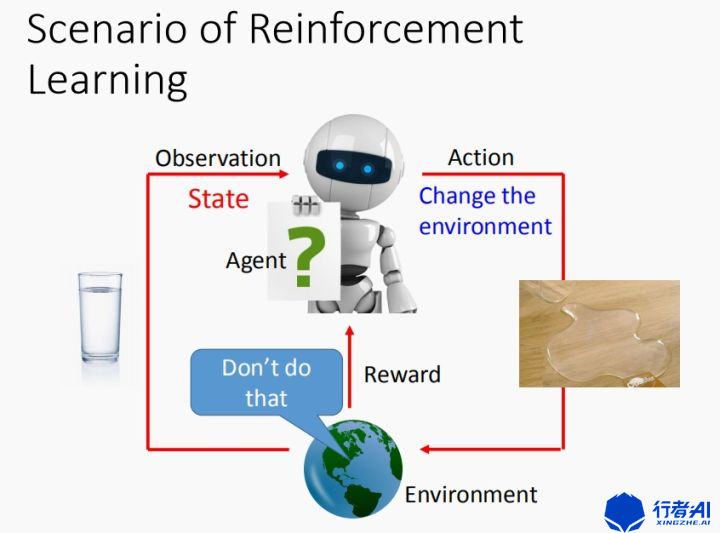Agent為智慧體，也就是我們的演算法，在遊戲當中以玩家的形式出現。智慧體通過一系列策略，輸出一個行為(Action)從而作用到環境(Environment)，而環境則返回作用後的狀態值也就是圖中的觀察(Observation)和獎勵值(Reward)。當環境返回獎勵值給智慧體之後，更新自身所在的狀態，而智慧體獲取到新的Observation。

### 1. ml-agents

<u>更多瞭解點選前往</u>

#### 1.2 Anaconda、tensorflow及tensorboard安裝

Tensorboard方便資料視覺化，方便分析模型是否達到預期。

#### 1.3 ml-agents安裝步驟

(1) 前往github下載ml-agents (本例項採用release6版本)

github可以下載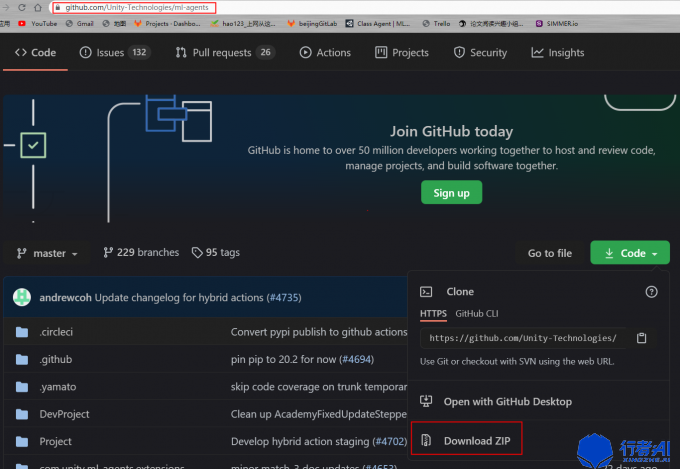(2) 將壓縮包解壓，把`com.unity.ml-agents``com.unity.ml-agents.extensions` 放入Unity的Packages目錄下(如果沒有請建立一個)，將`manifest.json`中加入此兩個目錄。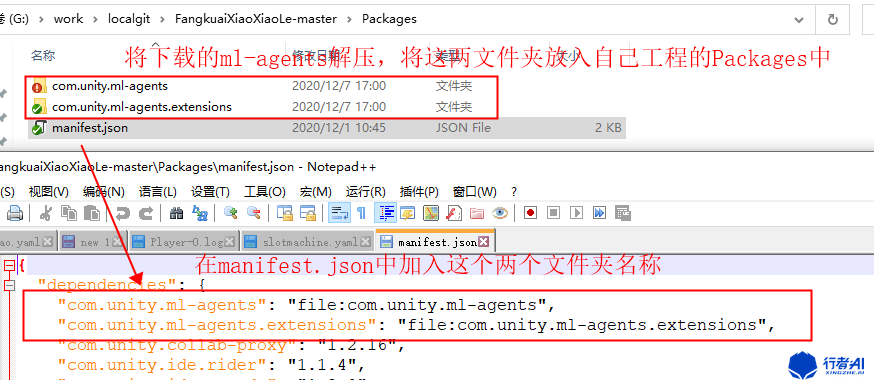(3) 安裝完成後，到工程中就匯入後，建立個新指令碼，輸入以下引用以驗證安裝成功

``````using Unity.MLAgents;
using Unity.MLAgents.Sensors;
using Unity.MLAgents.Policies;

public class MyAgent : Agent

{

}

``````

### 2. ml-agents訓練例項

#### 2.1 概要及工程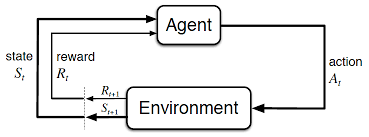Environment 通常利用馬爾可夫過程來描述，agent 通過採取某種 policy 來產生Action，和 Environment 互動，產生一個 Reward。之後 agent 根據 Reward 來調整優化當前的 policy。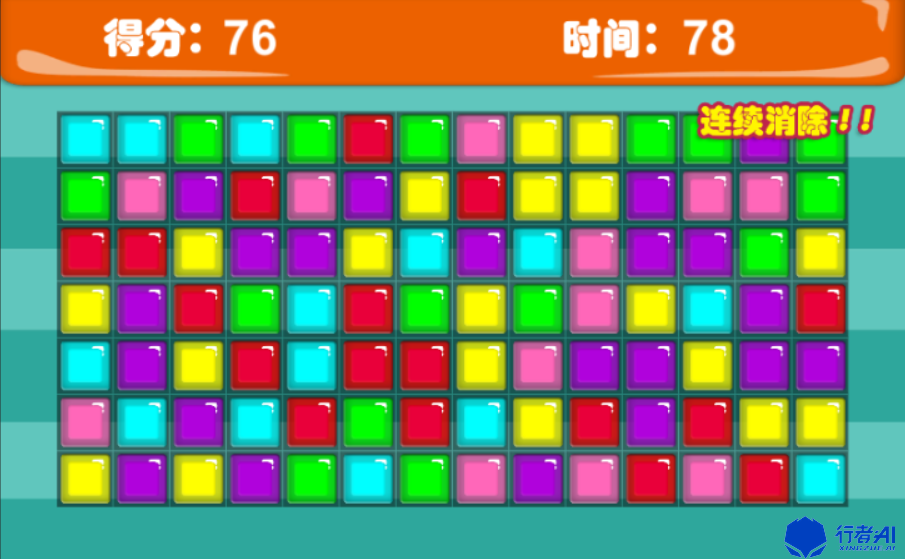Unity工程匯出部分請參考官方 點選前往

#### 2.2 遊戲框架AI介面抽離

``````static List<ML_Unit> states = new List<ML_Unit>();

public class ML_Unit
{
public int color = (int)CodeColor.ColorType.MaxNum;
public int widthIndex = -1;
public int heightIndex = -1;
}
//從當前畫面中，拿到所有方塊的資訊，包含所在位置x（長度）,位置y（高度）,顏色（座標軸零點在左上）
public static List<ML_Unit> GetStates()
{
states.Clear();
var xx = GameMgr.Instance.GetGameStates();
for(int i = 0; i < num_widthMax;i++)
{
for(int j = 0; j < num_heightMax; j++)
{
ML_Unit tempUnit = new ML_Unit();
try
{
tempUnit.color = (int)xx[i, j].getColorComponent.getColor;
}
catch
{
Debug.LogError(\$"GetStates i:{i} j:{j}");
}
tempUnit.widthIndex = xx[i, j].X;
tempUnit.heightIndex = xx[i, j].Y;
}
}
return states;
}

public enum MoveDir
{
up,
right,
down,
left,
}

public static bool CheckMoveValid(int widthIndex, int heigtIndex, int dir)
{
var valid = true;
if (widthIndex == 0 && dir == (int)MoveDir.left)
{
valid = false;
}
if (widthIndex == num_widthMax - 1 && dir == (int)MoveDir.right)
{
valid = false;
}

if (heigtIndex == 0 && dir == (int)MoveDir.up)
{
valid = false;
}

if (heigtIndex == num_heightMax - 1 && dir == (int)MoveDir.down)
{
valid = false;
}
return valid;
}

//執行動作的介面，根據位置資訊和移動方向，呼叫遊戲邏輯移動方塊。widthIndex 0-13,heigtIndex 0-6,dir 0-3 0上 1右 2下 3左
public static void SetAction(int widthIndex,int heigtIndex,int dir,bool immediately)
{
if (CheckMoveValid(widthIndex, heigtIndex, dir))
{
GameMgr.Instance.ExcuteAction(widthIndex, heigtIndex, dir, immediately);
}
}
``````

#### 2.3 遊戲AI演算法選擇

（本例使用PPO做演示，後續分享在ml-agents外接演算法，使用外部工具stable_baselines3，採用DQN的演算法來訓練）

#### 2.4 遊戲AI設計環境

--Observation：

ml-agents使用的swish作為啟用函式，可以使用不太大的浮點數(-10f ~10f)，但是為了讓agents獲得環境更純淨，訓練效果更理想，我們還是需要對環境進行編碼。

``````public class MyAgent : Agent
{
static List<ML_Unit> states = new List<ML_Unit>();
public class ML_Unit
{
public int color = (int)CodeColor.ColorType.MaxNum;
public int widthIndex = -1;
public int heightIndex = -1;
}

public static List<ML_Unit> GetStates()
{
states.Clear();
var xx = GameMgr.Instance.GetGameStates();
for(int i = 0; i < num_widthMax;i++)
{
for(int j = 0; j < num_heightMax; j++)
{
ML_Unit tempUnit = new ML_Unit();
try
{
tempUnit.color = (int)xx[i, j].getColorComponent.getColor;
}
catch
{
Debug.LogError(\$"GetStates i:{i} j:{j}");
}
tempUnit.widthIndex = xx[i, j].X;
tempUnit.heightIndex = xx[i, j].Y;
}
}
return states;
}

List<ML_Unit> curStates = new List<ML_Unit>();
public override void CollectObservations(VectorSensor sensor)
{
//需要判斷是否方塊移動結束，以及方塊結算結束
var codeMoveOver = GameMgr.Instance.IsCodeMoveOver();
{
return;
}

//獲得環境的狀態資訊
curStates = MlagentsMgr.GetStates();
for (int i = 0; i < curStates.Count; i++)
{
}
}
}
``````

--Action：

``````public class MyAgent : Agent
{
public enum MoveDir
{
up,
right,
down,
left,
}

public void DecomposeAction(int actionId,out int width,out int height,out int dir)
{
width = actionId / (num_heightMax * num_dirMax);
height = actionId % (num_heightMax * num_dirMax) / num_dirMax;
dir = actionId % (num_heightMax * num_dirMax) % num_dirMax;
}

//執行動作，並獲得該動作的獎勵
{
//需要判斷是否方塊移動結束，以及方塊結算結束
var codeMoveOver = GameMgr.Instance.IsCodeMoveOver();
{
return;
}

if (invalidNums.Contains((int)vectorAction))
{
//方塊結算的呼叫，這裡可以獲得獎勵（這裡是懲罰，因為這是在遮蔽動作內，訓練的時候會呼叫所有的動作，在非訓練的時候則不會進此邏輯）
GameMgr.Instance.OnGirdChangeOver?.Invoke(true, -5, false, false);
}
DecomposeAction((int)vectorAction, out int widthIndex, out int heightIndex, out int dirIndex);
//這裡回去執行動作，移動對應的方塊，朝對應的方向。執行完畢後會獲得獎勵，並根據情況重置場景
MlagentsMgr.SetAction(widthIndex, heightIndex, dirIndex, false);
}

//MlagentsMgr.SetAction呼叫後，執行完動作，會進入這個函式
public void RewardShape(int score)
{
//計算獲得的獎勵
var reward = (float)score * rewardScaler;
//將資料加入tensorboard進行統計分析
//每一步包含懲罰的動作，可以提升探索的效率
var punish = -1f / MaxStep * punishScaler;
//將資料加入tensorboard進行統計分析
}

{
// Mask the necessary actions if selected by the user.
checkinfo.Clear();
invalidNums.Clear();
int invalidNumber = -1;
for (int i = 0; i < MlagentsMgr.num_widthMax;i++)
{
for (int j = 0; j < MlagentsMgr.num_heightMax; j++)
{
if (i == 0)
{
invalidNumber = i * (num_widthMax + num_heightMax) + j * num_heightMax + (int)MoveDir.left;
}
if (i == num_widthMax - 1)
{
invalidNumber = i * (num_widthMax + num_heightMax) + j * num_heightMax + (int)MoveDir.right;
}

if (j == 0)
{
invalidNumber = i * (num_widthMax + num_heightMax) + j * num_heightMax + (int)MoveDir.up;
}

if (j == num_heightMax - 1)
{
invalidNumber = i * (num_widthMax + num_heightMax) + j * num_heightMax + (int)MoveDir.down;
}
}
}
}
}
``````

``````public class MyAgent : Agent
{
private void FixedUpdate()
{
var codeMoveOver = GameMgr.Instance.IsCodeMoveOver();
{
return;
}
//因為有協程需要等待時間，需要等待產生Reward後才去請求決策。所以不能使用ml-agents自帶的DecisionRequester
RequestDecision();
}
}

``````

#### 2.5 引數調整

``````behaviors:
SanXiaoAgent:
trainer_type: ppo
hyperparameters:
batch_size: 128
buffer_size: 2048
learning_rate: 0.0005
beta: 0.005
epsilon: 0.2
lambd: 0.9
num_epoch: 3
learning_rate_schedule: linear
network_settings:
normalize: false
hidden_units: 512
num_layers: 2
vis_encode_type: simple
memory: null
reward_signals:
extrinsic:
gamma: 0.99
strength: 1.0
init_path: null
keep_checkpoints: 25
checkpoint_interval: 100000
max_steps: 1000000
time_horizon: 128
summary_freq: 1000
self_play: null
behavioral_cloning: null
framework: tensorflow
``````

``````mlagents-learn config/ppo/sanxiao.yaml --env=G:\mylab\ml-agent-buildprojects\sanxiao\windows\display\121001display\fangkuaixiaoxiaole --run-id=121001xxl --train --width 800 --height 600 --num-envs 2 --force --initialize-from=121001
``````

``````(mlagents) PS G:\mylab\ml-agents-release_6> tensorboard --logdir=results --port=6006
TensorBoard 1.14.0 at http://PS20190711FUOV:6006/ (Press CTRL+C to quit)
``````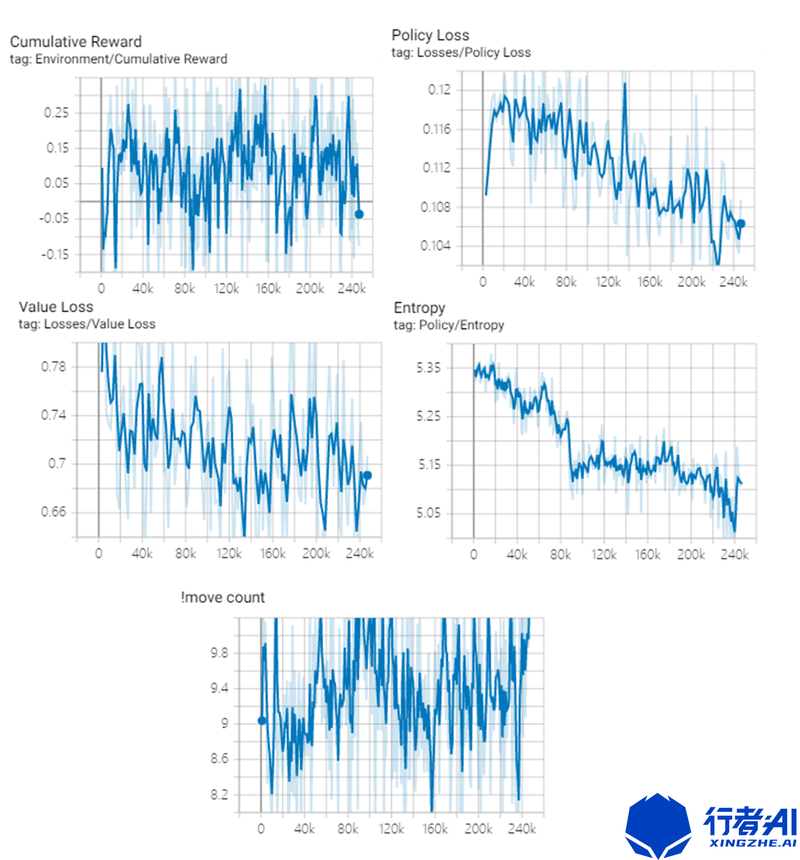–Reward：

``````//MlagentsMgr.SetAction呼叫後，執行完動作，會進入這個函式
public void RewardShape(int score)
{
//計算獲得的獎勵
var reward = (float)score * rewardScaler;
//將資料加入tensorboard進行統計分析
//每一步包含懲罰的動作，可以提升探索的效率
var punish = -1f / MaxStep * punishScaler;
//將資料加入tensorboard進行統計分析
}
``````

### 3. 總結及雜談

PS：更多技術乾貨，快關注【公眾號 | xingzhe_ai】，與行者一起討論吧！

「其他文章」